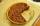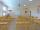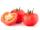Ratios

Divide:
a) 250 CZK in the ratio 2:3
b) 1000 CZK in the ratio 4:7:9

Result

a1 =  100
a2 =  150
b1 =  200
b2 =  350
b3 =  450

Solution:Leave us a comment of this math problem and its solution (i.e. if it is still somewhat unclear...):Be the first to comment!To solve this verbal math problem are needed these knowledge from mathematics:

Need help calculate sum, simplify or multiply fractions? Try our fraction calculator.

Next similar math problems:

1. AndreAndre, Thomas, and Ivan split 88 postage stamps in a 2:5:4 ratio. How much did Thomas get?
2. PointsGryffindor won 437 points. How many points obtained by each of the faculties if they were split at a ratio of 5: 7: 3: 4?
3. Ratio 11Simplify this ratio 10 : 1/4
4. Watching TVOne evening 2/3 students watch TV. Of those students, 3/8 watched a reality show. Of the students that watched the show, 1/4 of them recorded it. What fraction of the students watched and recorded reality tv.
5. PieVili ate three pieces of pie . If it piece is 1/8 how much pie did he eat?
6. Classroom 4In a class of 36 pupils, 2/3 are girls. How much it is in a class girls and boys?
7. Fractions 3Calculate 1/9 of 27:
8. Farmers 2On Wednesday the farmers at the Grant Farm picked 2 barrels of tomatoes. Thursday, the farmers picked 1/2 as many tomatoes as on Wednesday. How many barrels of tomatoes did the farmers pick on Thursday?
9. In fractionsAn ant climbs 2/5 of the pole on the first hour and climbs 1/4 of the pole on the next hour. What part of the pole does the ant climb in two hours?
10. WithdrawalIf I withdrew 2/5 of my total savings and spent 7/10 of that amount. What fraction do I have in left in my savings?
11. Lengths of the poolMiguel swam 6 lengths of the pool. Mat swam 3 times as far as Miguel. Lionel swam 1/3 as far as Miguel. How many lengths did mat swim?
12. Write 2Write 791 thousandths as fraction in expanded form.
13. ZdeněkZdeněk picked up 15 l of water from a 100-liter full-water barrel. Write a fraction of what part of Zdeněk's water he picked.
14. Mixed2improperWrite the mixed number as an improper fraction. 166 2/3
15. Fraction to decimalWrite the fraction 3/22 as a decimal.
16. Cupcakes 2Susi has 25 cupcakes. She gives 4/5. How much does she have left?
17. Passenger boatTwo-fifths of the passengers in the passenger boat were boys. 1/3 of them were girls and the rest were adult. If there were 60 passengers in the boat, how many more boys than adult were there?